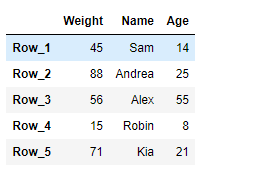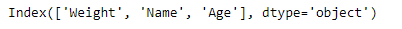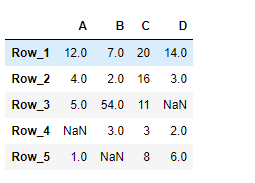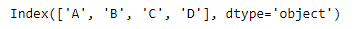# Python | Pandas DataFrame.columns

Pandas DataFrame is a two-dimensional size-mutable, potentially heterogeneous tabular data structure with labeled axes (rows and columns). Arithmetic operations align on both row and column labels. It can be thought of as a dict-like container for Series objects. This is the primary data structure of the Pandas.

Pandas` DataFrame.columns` attribute return the column labels of the given Dataframe.

Syntax: DataFrame.columns

Parameter : None

Returns : column names

Example #1: Use `DataFrame.columns` attribute to return the column labels of the given Dataframe.

 `# importing pandas as pd ` `import` `pandas as pd ` ` `  `# Creating the DataFrame ` `df ``=` `pd.DataFrame({``'Weight'``:[``45``, ``88``, ``56``, ``15``, ``71``], ` `                   ``'Name'``:[``'Sam'``, ``'Andrea'``, ``'Alex'``, ``'Robin'``, ``'Kia'``], ` `                   ``'Age'``:[``14``, ``25``, ``55``, ``8``, ``21``]}) ` ` `  `# Create the index ` `index_ ``=` `[``'Row_1'``, ``'Row_2'``, ``'Row_3'``, ``'Row_4'``, ``'Row_5'``] ` ` `  `# Set the index ` `df.index ``=` `index_ ` ` `  `# Print the DataFrame ` `print``(df) `

Output :Now we will use `DataFrame.columns` attribute to return the column labels of the given dataframe.

 `# return the column labels ` `result ``=` `df.columns ` ` `  `# Print the result ` `print``(result) `

Output :As we can see in the output, the `DataFrame.columns` attribute has successfully returned all of the column labels of the given dataframe.

Example #2: Use `DataFrame.columns` attribute to return the column labels of the given Dataframe.

 `# importing pandas as pd ` `import` `pandas as pd ` ` `  `# Creating the DataFrame ` `df ``=` `pd.DataFrame({``"A"``:[``12``, ``4``, ``5``, ``None``, ``1``],  ` `                   ``"B"``:[``7``, ``2``, ``54``, ``3``, ``None``],  ` `                   ``"C"``:[``20``, ``16``, ``11``, ``3``, ``8``],  ` `                   ``"D"``:[``14``, ``3``, ``None``, ``2``, ``6``]})  ` ` `  `# Create the index ` `index_ ``=` `[``'Row_1'``, ``'Row_2'``, ``'Row_3'``, ``'Row_4'``, ``'Row_5'``] ` ` `  `# Set the index ` `df.index ``=` `index_ ` ` `  `# Print the DataFrame ` `print``(df) `

Output :Now we will use `DataFrame.columns` attribute to return the column labels of the given dataframe.

 `# return the column labels ` `result ``=` `df.columns ` ` `  `# Print the result ` `print``(result) `

Output :As we can see in the output, the `DataFrame.columns` attribute has successfully returned all of the column labels of the given dataframe.

My Personal Notes arrow_drop_upCheck out this Author's contributed articles.

If you like GeeksforGeeks and would like to contribute, you can also write an article using contribute.geeksforgeeks.org or mail your article to contribute@geeksforgeeks.org. See your article appearing on the GeeksforGeeks main page and help other Geeks.

Please Improve this article if you find anything incorrect by clicking on the "Improve Article" button below.

Article Tags :

2

Please write to us at contribute@geeksforgeeks.org to report any issue with the above content.